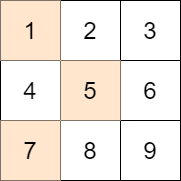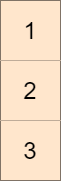1981. Minimize the Difference Between Target and Chosen Elements
Medium
739
125

You are given an `m x n` integer matrix `mat` and an integer `target`.

Choose one integer from each row in the matrix such that the absolute difference between `target` and the sum of the chosen elements is minimized.

Return the minimum absolute difference.

The absolute difference between two numbers `a` and `b` is the absolute value of `a - b`.

Example 1:```Input: mat = [[1,2,3],[4,5,6],[7,8,9]], target = 13
Output: 0
Explanation: One possible choice is to:
- Choose 1 from the first row.
- Choose 5 from the second row.
- Choose 7 from the third row.
The sum of the chosen elements is 13, which equals the target, so the absolute difference is 0.
```

Example 2:```Input: mat = [,,], target = 100
Output: 94
Explanation: The best possible choice is to:
- Choose 1 from the first row.
- Choose 2 from the second row.
- Choose 3 from the third row.
The sum of the chosen elements is 6, and the absolute difference is 94.
```

Example 3:```Input: mat = [[1,2,9,8,7]], target = 6
Output: 1
Explanation: The best choice is to choose 7 from the first row.
The absolute difference is 1.
```

Constraints:

• `m == mat.length`
• `n == mat[i].length`
• `1 <= m, n <= 70`
• `1 <= mat[i][j] <= 70`
• `1 <= target <= 800`
Accepted
20.2K
Submissions
62.2K
Acceptance Rate
32.4%

Seen this question in a real interview before?
1/4
Yes
No

Discussion (0)

Related Topics